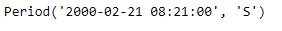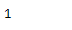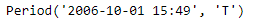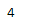# Python | Pandas Period.quarter

• Last Updated : 06 Jan, 2019

Python is a great language for doing data analysis, primarily because of the fantastic ecosystem of data-centric python packages. Pandas is one of those packages and makes importing and analyzing data much easier.

Pandas` Period.quarter` attribute return an integer value. The returned value represents the value of the quarter in the given period object.

Attention geek! Strengthen your foundations with the Python Programming Foundation Course and learn the basics.

To begin with, your interview preparations Enhance your Data Structures concepts with the Python DS Course. And to begin with your Machine Learning Journey, join the Machine Learning - Basic Level Course

Syntax : Period.quarter

Parameters : None

Return : integer value representing the value of quarter

Example #1: Use `Period.quarter `attribute to find the value of quarter in the given Period object.

 `# importing pandas as pd``import` `pandas as pd`` ` `# Create the Period object``prd ``=` `pd.Period(freq ``=``'S'``, year ``=` `2000``, month ``=` `2``,``                  ``day ``=` `21``, hour ``=` `8``, minute ``=` `21``)`` ` `# Print the Period object``print``(prd)`

Output :Now we will use the `Period.quarter` attribute to find out value of quarter in prd object.

 `# return value of quarter``prd.quarter`

Output :As we can see in the output, the `Period.quarter `attribute has returned 1 indicating that the given date in the prd object falls in the first quarter of the year.

Example #2: Use `Period.quarter` attribute to find the value of quarter in the given Period object.

 `# importing pandas as pd``import` `pandas as pd`` ` `# Create the Period object``prd ``=` `pd.Period(freq ``=``'T'``, year ``=` `2006``, month ``=` `10``,``                            ``hour ``=` `15``, minute ``=` `49``)`` ` `# Print the Period object``print``(prd)`

Output :Now we will use the `Period.quarter` attribute to find out value of quarter in prd object.

 `# return value of quarter``prd.quarter`

Output :As we can see in the output, the `Period.quarter `attribute has returned 4 indicating that the given date in the prd object falls in the fourth quarter of the year.

My Personal Notes arrow_drop_up